## Example Questions

← Previous 1

### Example Question #1 : Spheres

A cube with a surface area of 216 square units has a side length that is equal to the diameter of a certain sphere. What is the surface area of the sphere?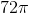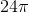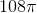Explanation:

Begin by solving for the length of one side of the cube. Use the formula for surface area to do this: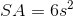s= length of one side of the cube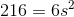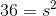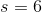The length of the side of the cube is equal to the diameter of the sphere. Therefore, the radius of the sphere is 3. Now use the formula for the surface area of a sphere: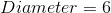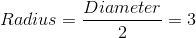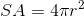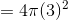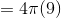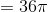The surface area of the sphere is.

### Example Question #1 : Spheres

The surface area of a sphere is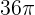. What is its diameter?Explanation:

The surface area of a sphere is defined by the equation:For our data, this means: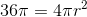Solving for, we get: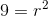or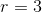The diameter of the sphere is.

### Example Question #3 : How To Find The Diameter Of A Sphere

The volume of one sphere is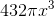. What is the diameter of a sphere of half that volume?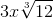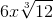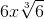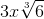Explanation:

Do not assume that the diameter will be half of the diameter of a sphere with volume of. Instead, begin with the sphere with a volume of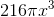Such a simple action will prevent a vexing error!

Thus, we know from our equation for the volume of a sphere that: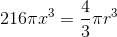Solving for, we get: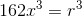If you take the cube-root of both sides, you have: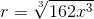First, you can factor out an: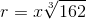Next, factor the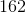: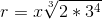Which simplifies to: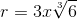Thus, the diameter is double that or:### Example Question #341 : Geometry

Find the surface area of a sphere with a diameter of 14. Use π = 22/7.

2464

428

872

616

1256

616

Explanation:

Surface Area = 4πr2 = 4 * 22/7 * 72 = 616

### Example Question #1 : How To Find The Surface Area Of A Sphere

A sphere has a surface area of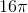square inches.  If the radius is doubled, what is the surface area of the larger sphere?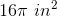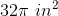Cannot be determined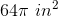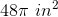Explanation:

The surface area of the larger sphere is NOT merely doubled from the smaller sphere, so we cannot doubleto find the answer.

We can use the surface area formula to find the radius of the original sphere.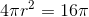r2 = 4

r = 2

Therefore the larger sphere has a radius of 2 * 2 = 4.

The new surface area is then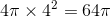square inches.

### Example Question #1531 : Gre Quantitative Reasoning

If a sphere has a volume ofcubic inches, what is its surface area?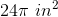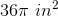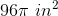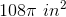Explanation:

The volume of a cube is equal to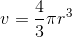.

So we mutiply our volume byand divide by, giving us.

The surface area of a sphere is equal to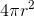, giving us.

### Example Question #4 : Spheres

How much does the volume of a sphere increase if its radius is increased by 50%?

50%

237.5%

150%

337.5%

0.3375%

237.5%

Explanation:

Recall the equation for the volume of a sphere:

V = (4/3)πr3

If we increase the radius by 50%, we can represent the new radius as being equal to r + 0.5r = 1.5r.

Replace this into the equation for the volume and simplify:

V2 = (4/3)π(1.5r)3 = (4/3)π(3.375r3)

Rewrite this so that you can compare the two volumes:

V= 3.375 * (4/3)πr3 = 3.375 * [(4/3)πr3]

This is the same as:

V2 = 3.375 * V

This means that the new volume is 337.5% of the original.  However, note that the question asked for the increase, which would be an increase by 237.5%.

### Example Question #5 : Spheres

A cube weighs 216 grams. If you carve a sphere out of the cube such that the diameter of the sphere is equal to one of the sides of the square, how many grams is the weight of the resulting sphere?

36π

288π

216π

144π

36π

Explanation:

Remember that the weight of an object is analogous to the volume. Since the weight of the sphere is 216, the volume of the sphere is proportional to 216. Remember the equation for volume of a sphere:

V = a * a * a = 216

Take the cube root of 216 to find that the length of one of the cubes is proportional to 6. According to the question, one of the sides of the cube is equivalent to the diameter of the sphere.

Thus d = 6 and r = d/2 = 3 for the sphere.

Remember the volume equation for a sphere:

V = 4/3 * π * r3

Plug in r = 3 to find V = 36π

### Example Question #1 : How To Find The Volume Of A Sphere

What is the volume of a sphere with a radius of 3?

36π

24π

96π

48π

112π

36π

Explanation:

Volume of a sphere = 4/3 * πr3 = 4/3 * π * 3= 36π

### Example Question #1 : How To Find The Volume Of A Sphere

How many times greater is the volume of a sphere with radius of 3 than the volume of a sphere with radius of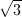?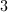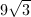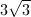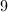Explanation:

The formula for the volume of a sphere is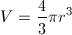.

Use this to find the volume of each sphere, then take the quotient of the two volumes to determine the relationship between the two.

For the first sphere,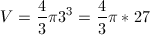.

For the second sphere,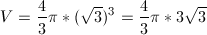.

Since both volumes have factors of 4/3 and pi, we'll ignore those and divide by the remaining factors. Keep in mind that the volume of the first sphere must be in the numerator due to the wording of the question.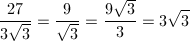← Previous 1

### All GRE Math Resources